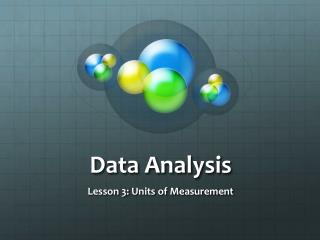DownloadDownload PresentationData Analysis

# Data Analysis

Télécharger la présentation## Data Analysis

- - - - - - - - - - - - - - - - - - - - - - - - - - - E N D - - - - - - - - - - - - - - - - - - - - - - - - - - -
##### Presentation Transcript

1. Data Analysis Lesson 3: Units of Measurement

2. International System of Units: the SI • The SI system: the metric system • The SI system is based on units of 10. • Base Units used in Chemistry • Seconds (s): time • Meters (m): length • Kilograms (kg): mass • Kelvin (K): temperature • Mole (mol): amount of a substance

3. SI Prefixes • Giga (G): 1,00o,000,000 • Mega (M): 1,000,000 • Kilo (K): 1,000 • Deci (d): 0.1 • Centi (c): 0.01 • Milli (m): 0.001 • Micro (μ): 0.000001 • Nano (n): 0.000000001

4. Derived SI Units • Derived units are those that occur due to combining two base units. • Volume: the space occupied by an object. • Length x width x height = cm3 • Liquid volumes are measured in liters (L). • One milliliter (mL) = 1 cm3 • Density is a ratio comparing mass to volume. • Grams/cubic centimeter • Grams/milliliter

5. Temperature: Celsius and Kelvin • Celsius scale • Anders Celsius invented a thermometer that was based on the temperatures at which water boils and freezes. • He set freezing at zero. • He set boiling at 100. • He then divided the distance between the two into one hundred equal units or degrees Celsius.

6. Kelvin scale • William Thomson, Lord Kelvin, devised the SI unit of temperature – the Kelvin. • Water freezes at 273 K • Water boils at 373 K • To convert from C to K, add 273. • To convert from K to C, subtract 273.